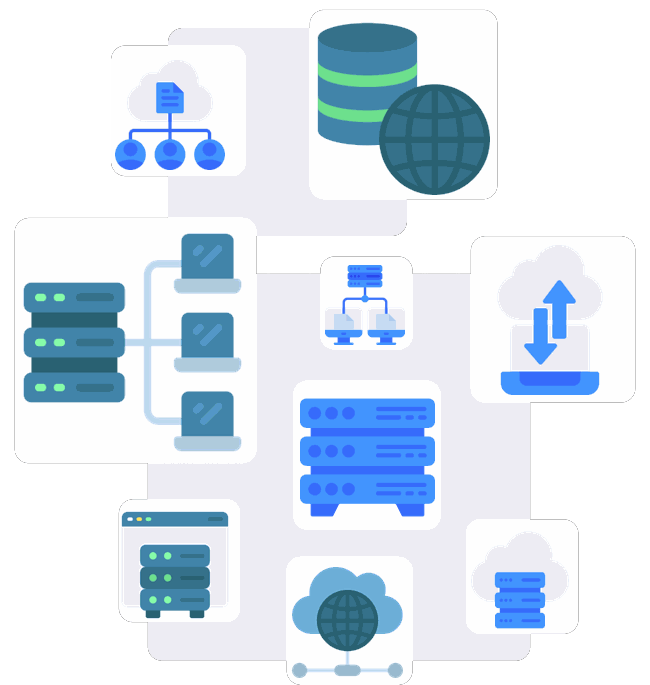# Numpy

Numpy (Numerical Python) is a library for the Python programming language that provides support for large multidimensional arrays and matrices, as well as a multitude of mathematical functions to perform operations on these arrays.## What is Numpy used for?

Numpy is used in a wide range of applications, including:

### Scientific and engineering calculations

Mathematical operations on arrays and matrices.

### Data processing

Cleaning, transforming, and analyzing data.

### Machine learning

Creating and training models based on data in array format.

## Technical aspects and capabilities of Numpy

### Multidimensional arrays

Numpy allows working with one-dimensional, two-dimensional, and multidimensional arrays, providing flexibility in structuring data.

### Functions for mathematical operations

Numpy includes a variety of functions for arithmetic, statistical, and trigonometric operations.

### Performance

Operations in Numpy are optimized and provide the ability to perform fast operations on large arrays.

### Integration with other languages

Interaction with data from other programming languages, such as C and Fortran.

## When to use Numpy?

### Data Volumes

Optimal for large multidimensional arrays.

Extensive set of functions for data processing.

### Performance

Faster than standard Python lists thanks to C-extensions.

### Compatibility

Integration with SciPy, Pandas, and scikit-learn.

For basic operations with Python data structures.

### Unstructured Data

Pandas may be preferable.

### Minimum Dependencies

For lightweight applications without additional libraries.

### Web Applications

Without the need for complex computations.

Ultimately, the choice to use or not to use Numpy depends on the specific needs of your project. Assess the tasks at hand and base your decision on their specifics.